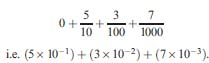Definition: decimal 2 from Merriam-Webster's Collegiate(R) Dictionary

(1651) : any real number expressed in base 10; esp : decimal fraction

Summary Article: decimal
From The Penguin Dictionary of Mathematics

A number expressed using the decimal number system. Commonly, the term is used for numbers that have fractional parts indicated by a decimal point. A number less than 1 is called a decimal fraction; for example, 0.537 is a way of writingA mixed decimal is one consisting of an integer and a decimal fraction (e.g. 27.63). The first position to the right of the point (representing tenths) is the first decimal place; the second position is the second decimal place; etc.

A decimal fraction is a series of fractions, i.e. it is a number of tenths plus a number of hundredths plus a number of thousandths, etc. The decimal may have a fixed number of digits: for example, 5/8 is 0.625; such numbers are called finite or terminating decimals. In other decimals the digits may continue indefinitely (they represent an infinite series); decimals of this type are called infinite or nonterminating decimals.

If the number is a rational number it may have an infinitely repeating digit or group of digits; decimals of this type are said to be repeating or recurring decimals.

Thus 1/3 is the decimal 0.333 33 …. This is sometimes written as 0.3 and referred to as ‘nought point three recurring’. Another example of a repeating decimal is 5/7, which is 0.714285 714… with the block of digits 714285 repeated endlessly; this is written as 0.714 285. Such decimals are also called periodic decimals. Irrational numbers, such as π, √2, and e, are decimals that are infinite but do not repeat; such numbers are termed nonrepeating or nonperiodic decimals.Copyright © Penguin Books Ltd, 1989, 1998, 2003, 2008

### Related Articles

##### Full text Article repetend
The Chambers Dictionary

the figure(s) that recur(s) in a recurring decimal number (maths); a recurring note, word, refrain, etc; anything that recurs or is repeated. adj

##### Full text Article decimal point
The Penguin Dictionary of Mathematics

A dot used to separate the integral part of a number from the fractional part in the decimal number system . The point is either centred (as in...

##### Full text Article Decimal system
Illustrated Dictionary of Science, Andromeda

A number system using the powers of ten; our everyday system of numeration. The digits used are 0, 1, 2, 3, 4, 5, 6, 7, 8, 9; the powers of 10...

See more from Credo